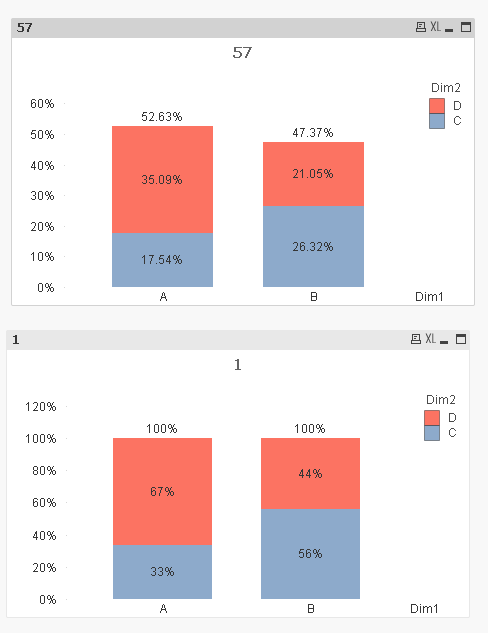# QlikView Creating Analytics

Discussion Board for collaboration related to Creating Analytics for QlikView.

Highlighted
Valued Contributor

## percentages on stacked chart

Hi ,

i have a stacked bar chart, where i want to display percentages insread of values on the data points.

Total bar value is 100% and every color represents its contribution.

PLease find the attached picture.

Tags (3)
1 Solution

Accepted SolutionsMVP

## Re: percentages on stacked chart

For two expression you can do this:

Expression1:

Expression1/RangeSum(Expression1, Expression2)

Expression2:

Expression2/RangeSum(Expression1, Expression2)

Replace Expression1 and Expression2 with your existing Expressions in your chart.

10 RepliesMVP

## Re: percentages on stacked chart

Assuming this is what your current expression is -? Sum(Sales), try changing it to this:

Sum(Sales)/Sum(TOTAL <FirstDimensionHere> Sales)

Valued Contributor

## Re: percentages on stacked chart

Did you try to check the relative in the expression?MVP

## Re: percentages on stacked chart

Relative with two dimensions is probably going to calculate the percentage based on the total which probably won't work for Mark

Valued Contributor

## Re: percentages on stacked chart

I may not understand what exactly you mean, however, I just tested out a simple example with 2 dimensions and it is based out the total of first dimension when checking relative.(which i think it is your set analysis means)MVP

## Re: percentages on stacked chart

See the difference between the two charts in the image belowTop image is using Relative and the bottom one uses this expression -> =Sum(Value)/Sum(TOTAL <Dim1> Value)

Script used:

Table:

LOAD * Inline [

Dim1, Dim2, Value

A, C, 10

A, D, 20

B, C, 15

B, D, 12

];

Valued Contributor

## Re: percentages on stacked chart

Sunny,

Really Thanq verymuch for your time and patience brother.

In my application, the stacked chart is comprised of 2 expressions.

I think that's the reason.

Let me share the application please.MVP

## Re: percentages on stacked chart

For two expression you can do this:

Expression1:

Expression1/RangeSum(Expression1, Expression2)

Expression2:

Expression2/RangeSum(Expression1, Expression2)

Replace Expression1 and Expression2 with your existing Expressions in your chart.

Valued Contributor

## Re: percentages on stacked chart

Thanq SunnyMVP

## Re: percentages on stacked chart

No problem at all Mark